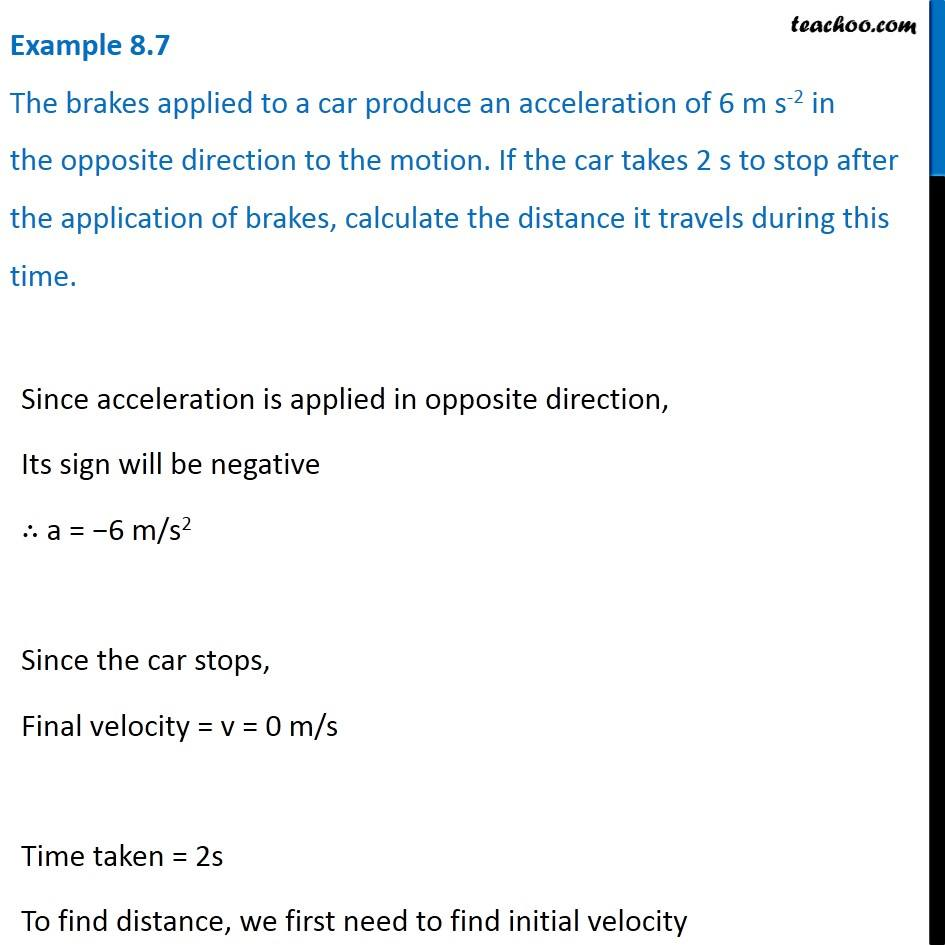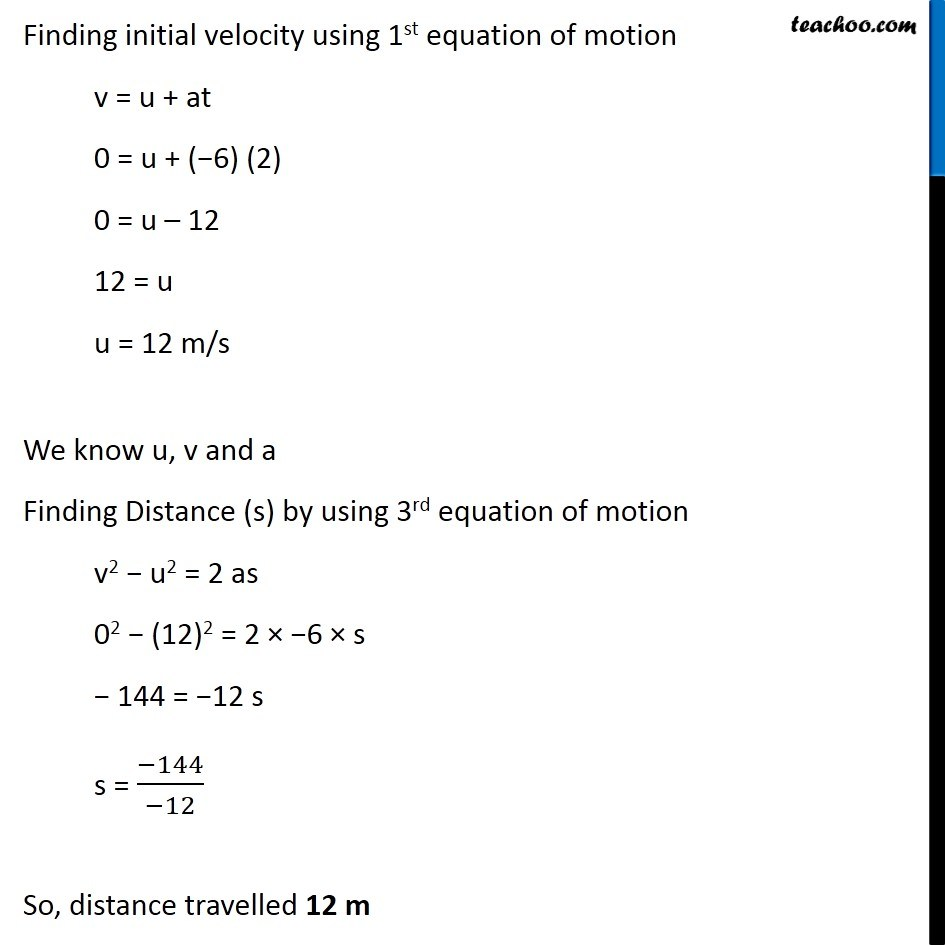Examples from NCERT Book

Class 9
Chapter 8 Class 9 - MotionLearn in your speed, with individual attention - Teachoo Maths 1-on-1 Class

### Transcript

Example 8.7 The brakes applied to a car produce an acceleration of 6 m s-2 in the opposite direction to the motion. If the car takes 2 s to stop after the application of brakes, calculate the distance it travels during this time. Since acceleration is applied in opposite direction, Its sign will be negative ∴ a = −6 m/s2 Since the car stops, Final velocity = v = 0 m/s Time taken = 2s To find distance, we first need to find initial velocity Finding initial velocity using 1st equation of motion v = u + at 0 = u + (−6) (2) 0 = u – 12 12 = u u = 12 m/s We know u, v and a Finding Distance (s) by using 3rd equation of motion v2 − u2 = 2 as 02 − (12)2 = 2 × −6 × s − 144 = −12 s s = (−144)/(−12) So, distance travelled 12 m